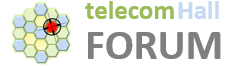# Introduction

If someone asks you “Which is the Signal Level for good call quality: -80 dbm or -90 dBm?”

Beware, if you respond quickly, you might end up missing something. This is because the correct answer is … it depends! The Signal Strength is a very important and essential measure for any technology (GSM, CDMA, UMTS, LTE, etc.). However, it is not the only one: let’s also talk a little about another magnitude, equally important: the Signal Noise Ratio.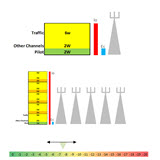Although this ratio is of fundamental importance to any cellular system, is not well understood by many professionals. On the opposite side, professionals with a good understanding of this ratio are able for example, to correctly assess the RF links, and also to perform more extensive optimizations, obtaining the best possible performance of the system.

So, let’s see a little about it?

# Eb and No

To begin, we define the basic concepts of Eb and No. They are basic for any digital communication system, and generally we talk about it when we deal with Bit Error Rate and also Modulation techniques.

Simply put:

• Eb : Bit Energy.

• It represents the amount of energy per bit.
• No : Noise Spectral Density.

• Unit: Watts/Hz (or mWatts/Hz)

Which brings us to the classic definition of Eb/No:

• Eb/No : Bit Energy on the Spectral Noise Density.

• Unit: dB

It didn’t help much, does it?

Do not worry. Indeed, only with the theoretical definition is still very difficult to see how this ratio is used, or how it can be measured.

But okay, let’s walk a little further.

# Okay, so how is Eb/No measured?

To understand how this ratio can be measured, let’s imagine a simple digital communication system.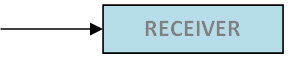The ratio Eb/No is measured at the receiver, and serves to indicate how strong the signal is.

Depending on the modulation technique used (BPSK, QPSK, etc.) we have different curves for Bit Error Rate x Eb/No.

These curves are used as follows: for a certain RF signal, which is the bit errors rate that I have? Is this bit error rate acceptable for my system?

Whereas the gain that digital has, then we can set a minimum criterion of signal to noise ratio, in order to have each service (Voice/Data) operating acceptably.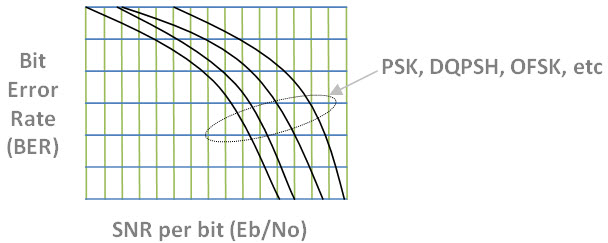In other words, we can theoretically determine how the performance would be for the digital link.

Note: it is worth remembering here that this is a very complex subject. As always, we try to introduce to you the most simplified possible through the use of examples and simple concepts. Okay?

For example, a concept that could be explored here - since we are talking about digital communication system - is the Noise Figure. But we do not want to repeat here all the theory explained in the University. Nor was it to have mentioned the noise figure here, but as we talked about it, just understand as a noise level that every receiver has, and that it is due to the process of amplification and processing of signal.

Concepts like this, and other even more complex, can be studied, if you wish. But now, let’s continue with our signal to noise ratio.

# Eb/No -> Ec/Io

The concept of Eb/No applies to any digital communication system. But today we are talking specifically to Ec/Io, which is a measure of evaluation and decisions of CDMA and UMTS.

Note: all the technology uses signal-interference ratio. For example, in GSM, we use C/I.

As we are speaking of codes, it becomes easier to understand the concepts by observing a simplified diagram of Spread Spectrum Modulation.

In red, in transmitter have a narrowband signal with data or voice modulated. This signal is spread and transmitted. And spreads through the middle (air). In the receiver, the signal is despread - using the same sequence that was spread - and thus recovering the base narrowband signal.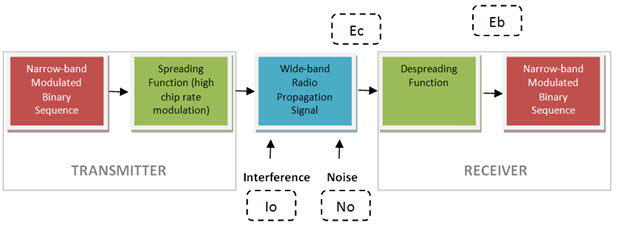To proceed, we must know some more definitions. However, this point is quite delicate, as we enter a conceptual area where we have differences between authors, differences in translations/countries, where differences in technologies are applied, etc…

Let’s try to define in a generic way, and only the main.

• No : Spectral Density of Noise;

• Noise generated by the RF components of the system, the air, among others.
• Io : Interference is the Broadband; Interfering co-channel, including yourself setor.

• E : is the signal (average) energy - do not confuse it with the sinal (average) power.

• b, c, s . …: Energy are the power points in time, therefore related to the measure or ‘length’ of the time (the average power is independent of time ).

• Hence it comes Eb, Ec and Es, respectively relating to Bit Chip and Symbol in different times.

Note: With these concepts, several formulas can be derived with different numerators and denominators. For example, Es = Eb * k, where k = number of bits per symbol. In QPSK modulation, where k = 2, Es = 2 * Eb. And the derivations of formulas can reach far more complex equations, such as the definitions of capacity of an AWGN channel, and further deductions for equivalences (Ec/No, Eb/Nt, etc. …). Again, it is not our purpose here today. We only mention a few concepts, related.

Then come back to the practical level - noting that theoretical approaches can be done more easily later, after the basics are understood.

So let’s keep today in ratios most common: Eb/No and Ec/Io.

As we defined Eb/No is the Average Energy of a bit signal, on the Spectral Density of Noise. It is primarily a parameter related to the manufacturer for different bearers (based on the channel model). But it can also vary with the environment (urban, rural, suburban), speed, diversity, use of power control, application type, etc…

And now we can begin to define Ec/Io , one of the most important systems in CDMA and UMTS.

Note: An important observation is that often when we refer to Ec/Io, we are actually referring to Ec/(Io + No). What happens is that for practical purposes, we only have Ec/Io, because the interference is much stronger and the noise can be neglected. Otherwise: for CDMA interference is like a noise, then both can be considered the same thing.

Okay, let’s stop with the issues and concepts, and talk a little about the values of these indicators and their use in practice.

# Positive Eb/No and Negative Ec/Io?

In terms of values, and talking logarithmicly, if any ratio is less than 1, then the value is negative. If greater than 1, positive.

We have Ec/Io in the air, which is spread across the spectrum: then we have negative value to the ratio of energy on the total noise (the energy is lower than the Total Interference). It is measured at the input of receiver (NodeB, UE, etc).

Regarding Eb/No, it is in the baseband after despreading and decoded only for one user - then we have a positive amount of energy over the total noise. It is measured at the output of receiver (NodeB, UE, etc).

# Why should we use Ec/Io?

A more natural question would be: why we can not simply use the Signal Strength measured by the mobile as a guide for operations such as handover?

The answer is simple: the measured signal level corresponds to the Total RF power - All cells that the mobile sees.

So we need another quick and simple measure that allows us to evaluate the contribution of each sector individually.

We used to measure the pilot channel signal of each sector to assess the quality: if the level of the pilot is good, then also are good levels for the traffic channels for our call in this sector. Likewise, if the pilot channel is degraded, so will the other channels (including traffic) be, and it is best to avoid using the traffic channels in this sector.

UMTS and CDMA systems, we have a pilot channel, some other control channels such as paging, and traffic channels.

The Ec/Io varies with several factors, such as the Traffic Load and and RF Scenario.

Of course, the Ec/Io is the final composition of all these factors simultaneously (Composite Ec/Io), but it’s easier to understand talking about each one separately.

# Change in Ec/Io according to the Sector Traffic Load

Each sector transmits a certain power. Suppose in our example we have a pilot channel power setting of 2 W, and a power of other control channels also fixed at 2 W.

To make it easier to understand, we calculate the Ec/Io (pilot channel power to total power) of this sector in a situation where we have no busy traffic channel (0 W).Thus we have:

Ec = 2 W

Io = 0 + 2 + 2 = 4 W

Ec/Io = (2/4) = 0.5 = -3 dB

Now assume that several traffic channels are busy (eg use 6 W for traffic channels). This is a situation of traffic load, we’ll see how is Ec/Io.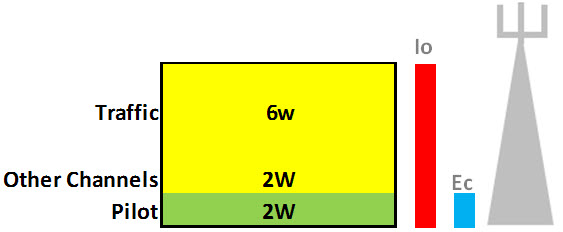Ec = 2 W

Io = 2 + 2 + 6 = 10 W

Ec/Io = (2/10) = 0.2 = -7 dB

Conclusion : As the traffic load in the sector increases, the Ec/Io worsens.

# Change in Ec/Io according to the RF scenario

According to the RF scenario - a single server sector, some or many servers sectors - we can also take various measures to Ec/Io.

Considering first a situation without external interference, with only one server sector (dominant), the ratio Ec/Io is about the same initially transmitted.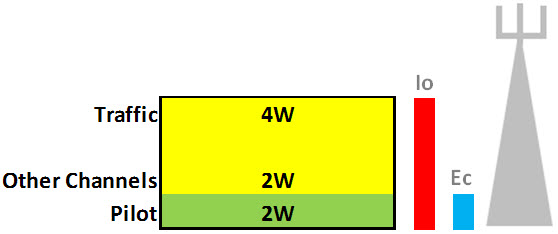Ec/Io = (2/8) = 0.25 = -6 dB

Whereas a signal coming from this sector in the mobile at level of -90 dBm (Io = -90 dBm), we have Ec = -90 dBm + (- 6 db) = -96 dBm.

Let us now consider another situation. Instead of one, we have five sectors signal arriving at the mobile (for simplicity, all with the same level of -90 dBm).Now have Io = -83 dBm (which is the sum of five signals of -90 dBm). And the power of our pilot channel remains the same (Ec = -96 dBm).

Thus: Ec/Io = -96 - (-83) = -13 dB

Conclusion : As many more sectors serves the mobile, the Ec/Io worsens.

This situation where we have many overlapping sectors, and with the same level of signal is known as Pilot Pollution - the mobile sees them all at once - each acting as interferer to each other.

The solution in such cases is to eliminate unwanted signals, by setting power parameters or physical adjustments (tilt, azimuth), leaving just dominant signals which should exist at this problematic place.

# Okay, and what are typical values?

We have seen that for CDMA and UMTS systems, the measurement of Ec/Io which is very important in the analysis, especially in handover decisions.

And now also understand the measure Ec/Io as the ratio of ‘good’ energy over ‘bad’ energy, or ‘cleaness’ of signal.

But what are the practical values?

The value of Ec/Io fluctuates (varies), as well as any wireless signal. If the value starts to get too low, you start to have dropped calls, or can not connect. But what then is a good range of Ec/Io for a sign?

In practical terms, values of Ec/Io for a good evaluation of the network (in terms of this indicator) are shown in the diagram below.A composite Ec/Io ~ - 10 db is a reasonable value to consider as good.

Note: See we are talking about negative values, and considering them ‘good’. In other words, we are saying that energy is below the Noise (and still have a good situation).

This is a characteristic of the system itself, and Ec/Io ‘most negative’ or ‘less negative’ is going to allow assessment of the communication.

In situations where Ec/Io is very low (high negative number), and the signal level too (also high negative number), first we need to worry in enhancing the weak signal.

Another typical situation: if the measured Ec/Io is very low, even if you have a good signal level, you can not connect, or the call will drop constantly.

I hope you’ve managed to understand how the Ec/Io is important for CDMA and UMTS. Note, however, that this matter is very complex, and supplementary reading - books and internet - can further help you become an expert on the subject.

Anyway, the content displayed serves as an excellent reference, especially if you’re not familiar with the concept of signal over noise for CDMA and UMTS.

# And the Signal to Noise Ratio for other technologies?

The ratio Ec/Io is the most commonly used to assess the condition of energy over interference, but applies only in technologies that use codes (Ec).

But the concepts understood here to CDMA and UMTS are very similar - apply - for any technology, eg GSM, where we use the C/I.

Anyway, this is a topic for another tutorial, we saw today Ec/Io.

# Conclusion

Today we had a brief introduction on the Ec/Io ratio, a measurement for decisions in CDMA and UMTS, and used togheter with the measured Signal Strength.

We have seen that it represents the ratio of signal energy within the duration of a chip of the pilot channel, on the Spectral Density of Noise + Interference.

This is a very important measure, which somehow ignores the overall strength of the signal, and focuses on how best to evaluate the pilot channel signal is desired, in relation to noise that interferes with it.

Returning to our original question: A strong signal level does not necessarily indicate an strong Ec/Io: it depends on the interference level!

telecomHall CommunityTelecom Made Easy!(Share to your friends)